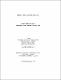## Energy of graphs and digraphs2010
##### Authors
Jahanbakht, Nafiseh
University of Lethbridge. Faculty of Arts and Science
##### Publisher
Lethbridge, Alta. : University of Lethbridge, Dept. of Mathematics and Computer Science, c2010
##### Abstract
The energy of a graph is the sum of the absolute values of the eigenvalues of its adjacency matrix. The concept is related to the energy of a class of molecules in chemistry and was first brought to mathematics by Gutman in 1978 (). In this thesis, we do a comprehensive study on the energy of graphs and digraphs. In Chapter 3, we review some existing upper and lower bounds for the energy of a graph. We come up with some new results in this chapter. A graph with n vertices is hyper-energetic if its energy is greater than 2n−2. Some classes of graphs are proved to be hyper-energetic. We find a new class of hyper-energetic graphs which is introduced and proved to be hyper-energetic in Section 3.3. The energy of a digraph is the sum of the absolute values of the real part of the eigenvalues of its adjacency matrix. In Chapter 4, we study the energy of digraphs in a way that Pe˜na and Rada in  have defined. Some known upper and lower bounds for the energy of digraphs are reviewed. In Section 4.5, we bring examples of some classes of digraphs in which we find their energy. Keywords. Energy of a graph, hyper-energetic graph, energy of a digraph.
##### Description
vii, 80 leaves ; 29 cm
##### Keywords
Graph theory , Directed graphs , Eigenvalues , Dissertations, Academic